SEARCH HOMEMath Central Quandaries & QueriesSubject: Octagonal Bird House Roof Angle Cuts Name: Soren Who are you: Other I'm in the process of building a birdhouse that is an octagon (based on previous questions, looks like that's a familiar tune). The essential elements are known, but I get stuck when trying to determine the angle for the cuts that would be made to the thickness of the wood so that they all fit together when assembled. Each octagonal section is 7 inches in width and the peak of the roof will be 2 inches higher than the sides. My sense is that the angle cuts that need to be made to the 'height' of each piece of wood. By height I mean the thinnest part of the wood that is neither the length nor the width to use colloquial terms. While it's clear that a slight angle is needed, it would seem that the angle would necessarily change as the distance from the top of any one side to the peak changes. Please advise if more clarification is needed. The 2 inches is random and can be changed if more convenient. Whew! Thank you.Soren,

I drew a (very crude and not to scale) diagram of what I think is the design of your birdhouse.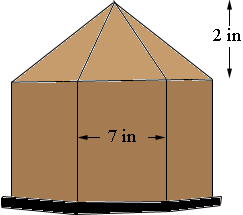then drew a cross section showing a side and a piece of the roof. Is the angle A the angle you want?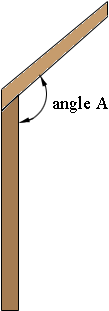Harley

Soren wrote back.

Hello Harley -

Thank you for getting back to me so quickly. I had a feeling that I didn't articulate the question very well.

Having 'acquired' this image from another web site, assume for a moment that we're looking at one of the eight roofing sections (as the question is strictly limited to the roofing sections themselves, and not with the fit between the roof and birdhouse itself). Looking at the image, I'm thinking of the cut that needs to be made down 'side b'.

Here's what I'm thinking but I could be way off, but here goes. Assume for the purposes of discussion that the roof of the bird house is totally flat but that we're going through the exercise of making one that needs eight sections anyhow. Side b would require no angled cut down the side to allow for a tight fit because the whole thing is flat. However, as the peak of the roof rises (e.g. the center of the roof goes up), I'm assuming that an angled cut would need to be made for a tight fit so that all pieces would fit snugly together on the roof. Otherwise the depth of the pieces would align on the inside, but not outside the roof. Now I could just cut a 15-degree angle on these since the very tops would fit anyway, but now I'm curious as to how the heck to figure this out.

In short, if you add the third dimension to the drawing below that would create the thickness of the wood, I'm trying to determine what angle that thickness needs to be cut at so that the pieces fit snugly together both inside and out.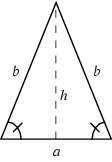Hope that helps!!!
Soren

Hi Soren,

I think I understand now.

I used vectors to approach your problem and Mathematica to perform the calculations.

In the diagram below of the roof of the birdhouse I have drawn vectors v1, v2 ,v3 and v4 along the edges of two adjacent triangular sections.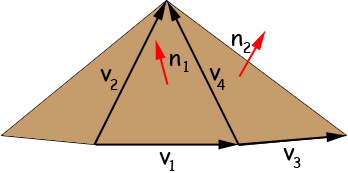The cross product of the vectors v1 and v2 gives a vector n1 which is perpendicular (normal) to the roof section containing the vectors v1 and v2. Likewise the cross product of the vectors v3 and v4 gives a vector n2 which is perpendicular (normal) to the roof section containing the vectors v3 and v4. The angle between the adjacent roof sections is then the angle between the vectors n1 and n2 and thus the angle of the cut will be half this angle.

To find the vectors I am going to impose a coordinate system with the origin at the initial point of v1 and v2 , with v1 along the x-axis, the y-axis pointing into the page and the z-axis vertical.

Here is a cross section of the birdhouse at the base of the roof. v1 = OP and v3 = PQ.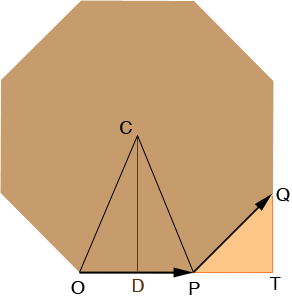I let s be the length of a side of the octagon (the distance from O to P) and h be the height of the roof section of the birdhouse. The coordinates of O are (0,0,0) and the coordinates of P are (s,0,0). The angle PCO is 360/8 = 45 degrees or π/4 radians. (I am going to use radians for the angle measurements sinceMathematica uses radians.) The sum of the angles in a triangle is 180 degrees (π radians) and the triangle COP is isosceles, hence the angle COP is 3/8 π radians. D is the midpoint of OP and the angle ODC is a right angle and hence tan(COD) = |DC|/|OD|. Thus the y-coordinate of C is

|CD| = s/2 tan( 3/8 π).

Thus the coordinates of the point at the peak of the roof are (s/2, s/2 tan( 3/8 π), h).

Triangle QPT is a right triangle with angles QPT and TQP each measuring π/4 radians (45 degrees) and |PQ| = s and thus |PT| = |TQ| = s/√2 and hence Q has coordinates (s + s/√2, s/√2, 0).

From this information I can find the vectors I need

v1 = (s,0,0)
v2 = (s/2, s/2 tan( 3/8 π), h)
v3 = (s/√2, s/√2, 0) and
v4 = (-s/2, s/2 tan( 3/8 π), h)

This will allow me to find n1 and n2 .

The dot product of n1 and n2 is given by

n1· n2 = |n1| |n2| cos(theta)

where |n1| is the length of n1 , |n2| is the length of n2 and theta is the angle between them. Thus

cos(theta) = n1· n2/( |n1| |n2|) and theta = arccos( n1· n2/( |n1| |n2|)).

Now I am ready to use Mathematica.

Below is the output from my Mathematica session.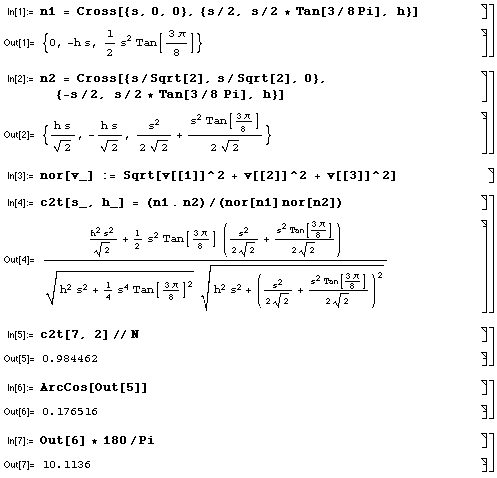Cross is the cross product function, nor is a function to calculate the length of a vector in 3-space, and c2t is the cos(theta) function defined above. I then calculated this function for the values you gave, s = 7 inches and h = 4 inches. The arccos function gave an angle of 0.176516 radians which I then converted to 10.1136 degrees. Thus you need to set your saw blade to 10.1136/2 = 5 degrees.

HarleyMath Central is supported by the University of Regina and The Pacific Institute for the Mathematical Sciences.